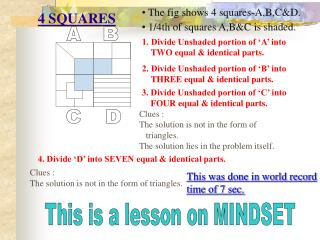DownloadDownload Presentation4 SQUARES

# 4 SQUARES

Télécharger la présentation## 4 SQUARES

- - - - - - - - - - - - - - - - - - - - - - - - - - - E N D - - - - - - - - - - - - - - - - - - - - - - - - - - -
##### Presentation Transcript

1. 4 SQUARES • The fig shows 4 squares-A,B,C&D. • 1/4th of squares A,B&C is shaded. A B 1. Divide Unshaded portion of ‘A’ into TWO equal & identical parts. 2. Divide Unshaded portion of ‘B’ into THREE equal & identical parts. 3. Divide Unshaded portion of ‘C’ into FOUR equal & identical parts. Clues : The solution is not in the form of triangles. The solution lies in the problem itself. C D 4. Divide ‘D’ into SEVEN equal & identical parts. Clues : The solution is not in the form of triangles. This was done in world record time of 7 sec. This is a lesson on MINDSET